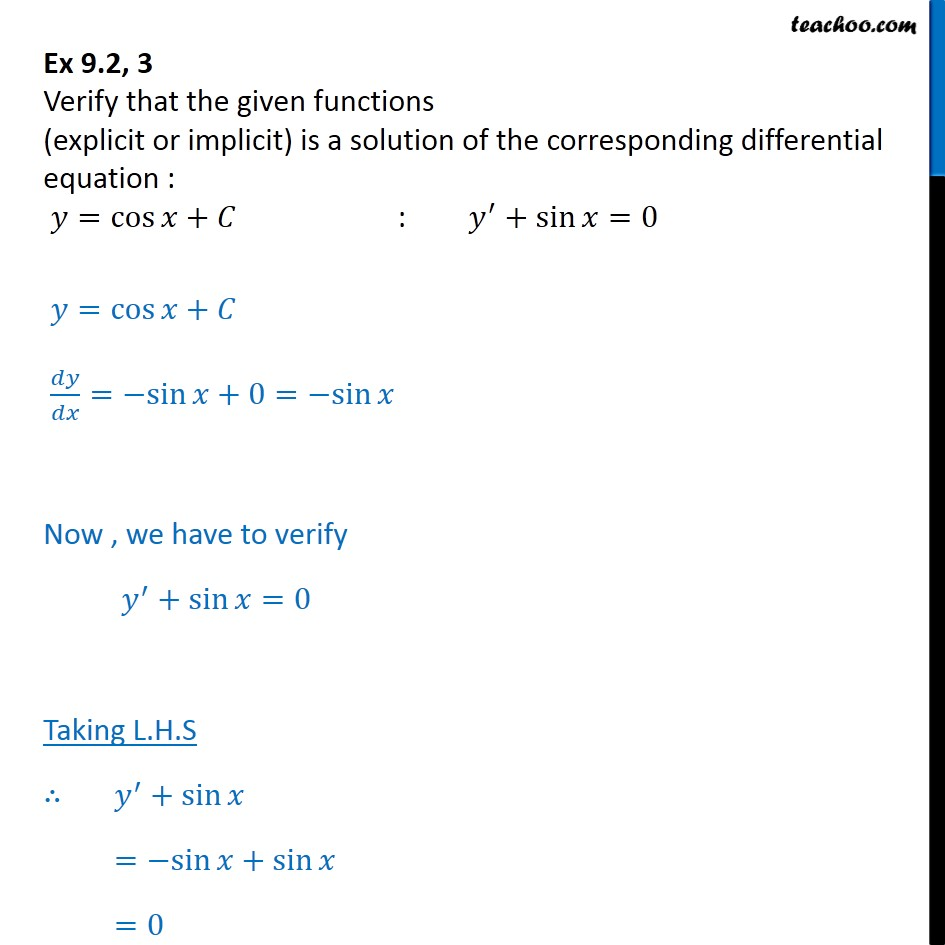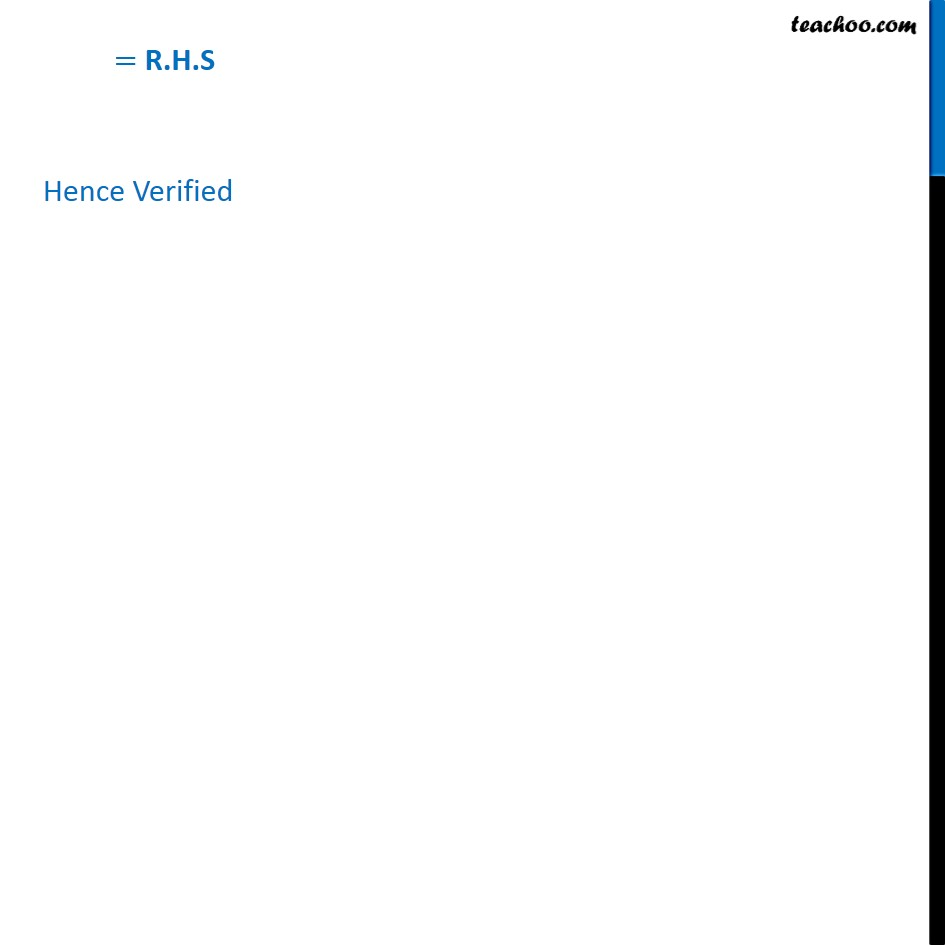Ex 9.2

Chapter 9 Class 12 Differential Equations
Serial order wiseLearn in your speed, with individual attention - Teachoo Maths 1-on-1 Class

### Transcript

Ex 9.2, 3 Verify that the given functions (explicit or implicit) is a solution of the corresponding differential equation : 𝑦= cos﷮𝑥+𝐶﷯ : 𝑦﷮′﷯+ sin﷮𝑥=0﷯ 𝑦= cos﷮𝑥+𝐶﷯ 𝑑𝑦﷮𝑑𝑥﷯=−sin⁡𝑥+0=−sin⁡𝑥 Now , we have to verify 𝑦﷮′﷯+sin⁡𝑥=0 Taking L.H.S ∴ 𝑦﷮′﷯+sin⁡𝑥 =−sin⁡𝑥+sin⁡𝑥 =0 = R.H.S Hence Verified